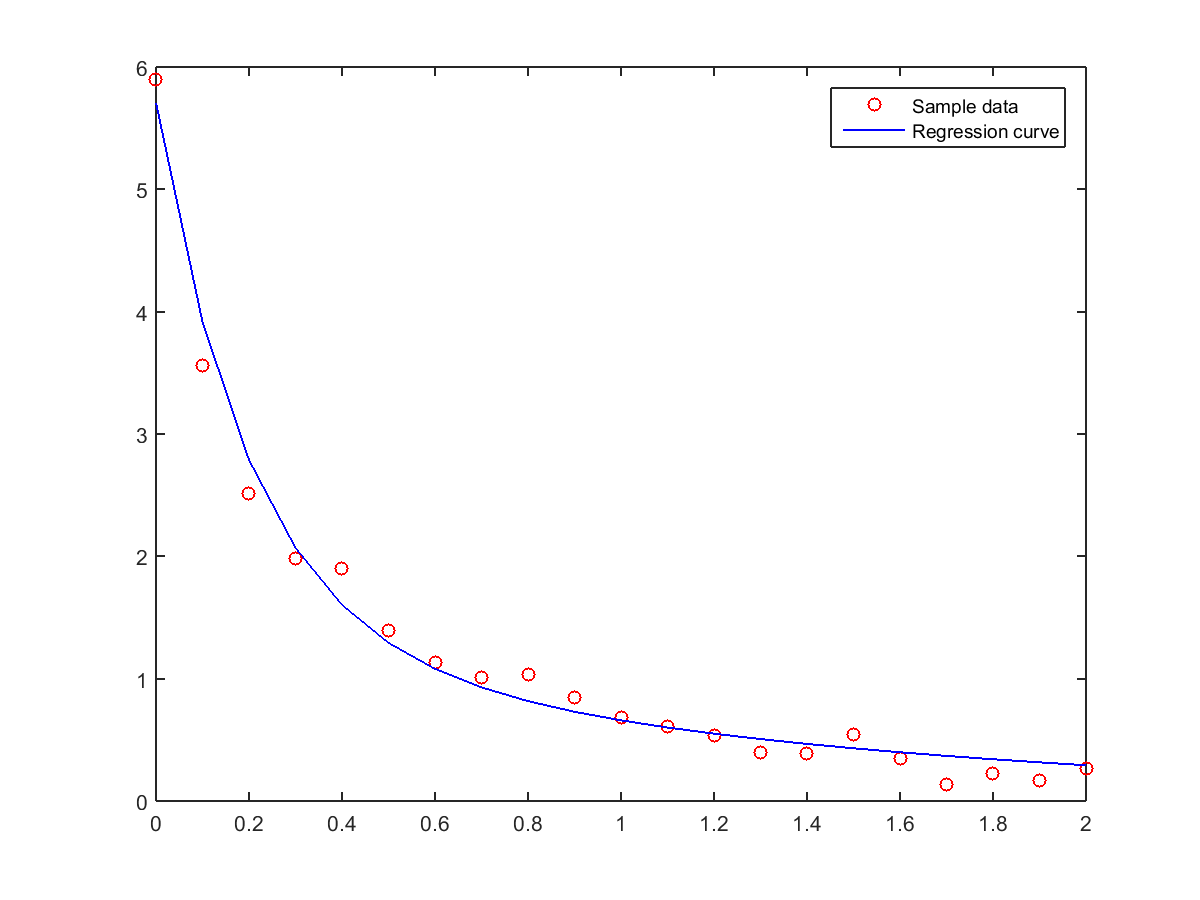## 10-3 嚙踝蕭嚙踝蕭嚙踝蕭嚙踝蕭嚙踝蕭嚙踝蕭嚙踝蕭嚙踝蕭嚙踝蕭嚙踝蕭嚙踝蕭嚙踝蕭 fminsearch

• 無法一次找到最佳解。
• 無法保證能夠找到最佳解。
• 須引用各種非線性最佳化的方法。
• 各種相關數學性質並不明顯。

$$y= a_1 e^{\lambda_1 x} + a_2 e^{\lambda_2 x}$$

Example 1: 10-曲線擬合與迴歸分析/errorMeasure1.mfunction squaredError = errorMeasure1(theta, data) if nargin<1; return; end x = data(:,1); y = data(:,2); y2 = theta(1)*exp(theta(3)*x)+theta(2)*exp(theta(4)*x); squaredError = sum((y-y2).^2);

Example 2: 10-曲線擬合與迴歸分析/nonlinearFit01.mload data.txt theta0 = [0 0 0 0]; tic theta = fminsearch(@(x)errorMeasure1(x, data), theta0); fprintf('計算時間 = %g\n', toc); x = data(:, 1); y = data(:, 2); y2 = theta(1)*exp(theta(3)*x)+theta(2)*exp(theta(4)*x); plot(x, y, 'ro', x, y2, 'b-'); legend('Sample data', 'Regression curve'); fprintf('誤差平方和 = %d\n', sum((y-y2).^2));計算時間 = 0.0762827 誤差平方和 = 5.337871e-01MATLAB程式設計：進階篇